Author: Oscar Cronquist Article last updated on May 14, 2022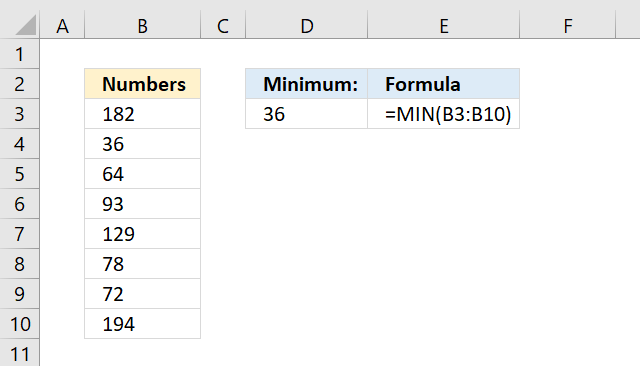The MIN function allows you to retrieve the smallest number in a cell range.

The formula in cell D3 extracts the smallest number in cell range B3:B10, see picture above.

## 1. MIN Function Syntax

MIN(number1, [number2], ...)

## 2. MIN Function Arguments

 Argument Text number1 You need at least one argument. Required. [number2] Up to 254 arguments are possible. Optional.

## 3. MIN function example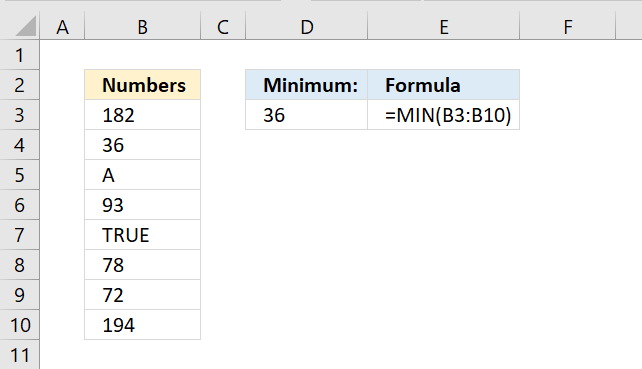The image above shows the MIN function in cell D3, cell range B3:B10 contains random text, numbers, and boolean values. The MIN function ignores text and boolean values, however not errors.

Formula in cell E3:

=MIN(B3:B10)

Arguments can be constants, named ranges, cell references, and arrays.

MIN(B3:B10)

becomes

MIN({182; 36; "A"; 93; TRUE; 78; 72; 194})

and returns 36.

## 4. MIN function ignore zero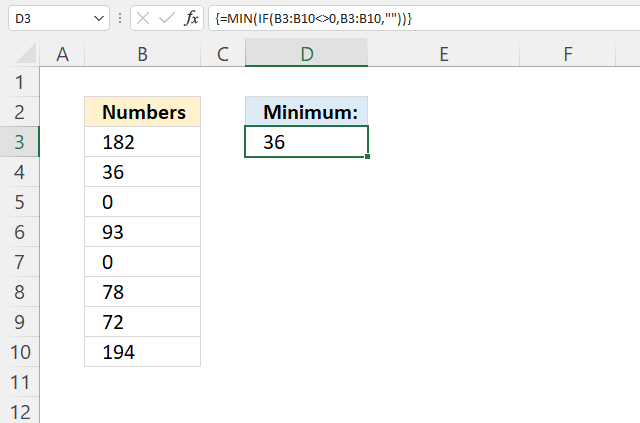The image above demonstartes a formula that extracts the smallest number in a given cell range ignoring zeros.

Array formula in cell D3:

=MIN(IF(B3:B10<>0, B3:B10, ""))

### 4.1 How to enter an array formula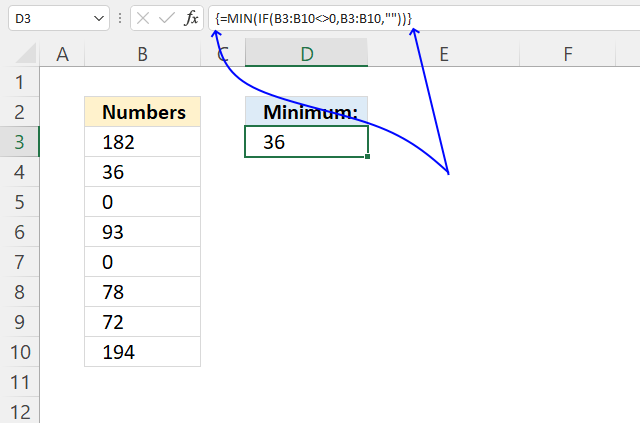1. Copy the array formula above.
2. Double press with the left mouse button on cell D3, a prompt appears.
3. Paste it to cell D3, shortcut keys are CTRL + v.
4. Press and hold CTRL + SHIFT keys simultaneously.
5. Press Enter once.
6. Release all keys.

The formula bar shows a beginning and ending curly bracket, don't enter these characters yourself.

They appear automatically if you followed the above steps.

Note, Excel 365 users can ignore these steps. Array formulas are entered like regular formulas in Excel 365.

### 4.2 Explaining formula

#### Step 1 - Find numbers not equal to zero

The less than and larger than signs combined evaluates to not equal to, the result is a boolean value TRUE or FALSE.

B3:B10<>0

becomes

{182; 36; 0; 93; 0; 78; 72; 194}<>0

and returns

{TRUE; TRUE; FALSE; TRUE; FALSE; TRUE; TRUE; TRUE}.

#### Step 2 - Replace TRUE with nothing

The IF function returns one value if the logical test is TRUE and another value if the logical test is FALSE.

IF(logical_test, [value_if_true], [value_if_false])

IF(B3:B10<>0, B3:B10, "")

becomes

IF({TRUE; TRUE; FALSE; TRUE; FALSE; TRUE; TRUE; TRUE}, B3:B10, "")

becomes

IF({TRUE; TRUE; FALSE; TRUE; FALSE; TRUE; TRUE; TRUE}, {182; 36; 0; 93; 0; 78; 72; 194}, "")

and returns {182; 36; ""; 93; ""; 78; 72; 194}.

#### Step 3 - Extract smallest number in array

MIN(IF(B3:B10<>0, B3:B10, ""))

becomes

MIN({182; 36; ""; 93; ""; 78; 72; 194})

and returns 36.

## 5. MIN function returns 0 (zero)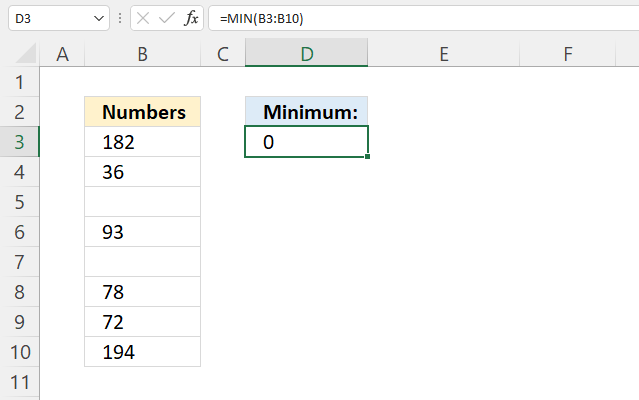The image above shows a scenario where the MIN function returns zero and the data source contains no zeros, at least, that is what it looks like.

Formula in cell D3:

=MIN(B3:B10)

Cell B3:B10 contains blank cells but when we check the cell formatting dialog box zeros have been hidden. See the image below.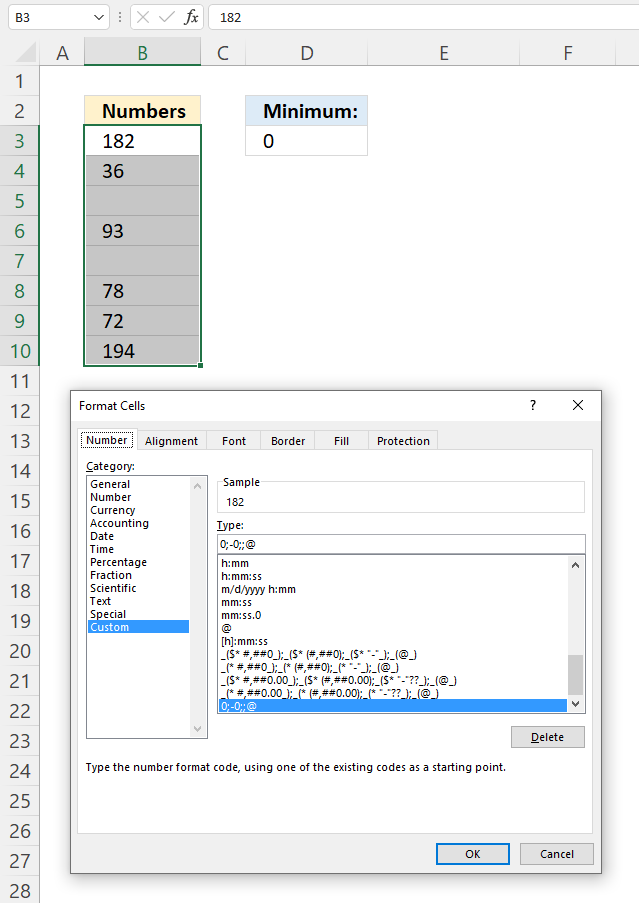Here is how to check if zeros are hidden.

1. Select your data. Cell range B3:B10 in this example.
2. Press CTRL + 1 to open the "Format Cells" dialog box.
3. Select category "General".
4. Press with the left mouse button on the "OK" button to apply changes.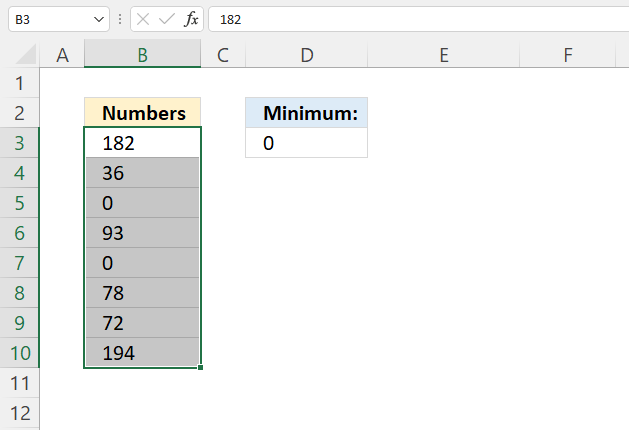Check the Excel options if this is not working for you. Here is how to do that in Excel 365:

1. Press with the left mouse button on tab "File" on the ribbon.
2. Press with left mouse button on "Options".
3. Go to "Advanced", see the image below.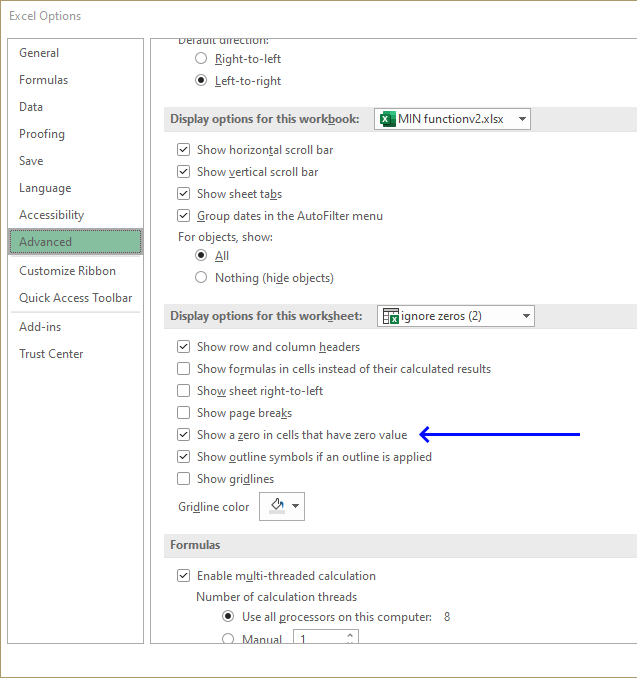4. Scroll down until you find "Show a zero in cells that have zero value". Make sure the checkbox is enabled.
5. Press with the left mouse button on the "OK" button to apply changes.

Check the next section if this is also not a solution for you.

## 6. MIN function not working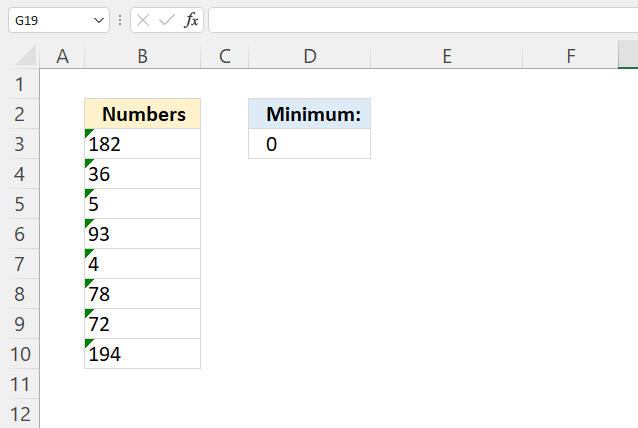This example demonstrates numbers stored as text may make the MIN function return 0 (zero). Numbers stored as text are left-aligned (default), a green arrow in the top left corner is an indication of numbers stored as text.

Formula in cell D3:

=MIN(B3:B10)

Here is how to convert numbers stored as text.

1. Select the data, this is cell range B3:B10 in the example above.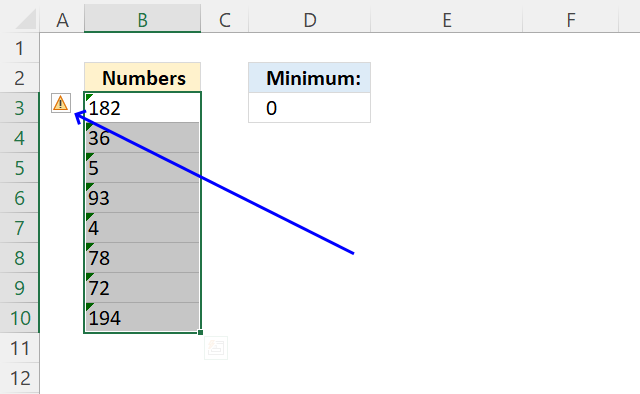2. An "exclamation" sign appears.
3. Press with left mouse button on the "Exclamation" sign to open a context menu.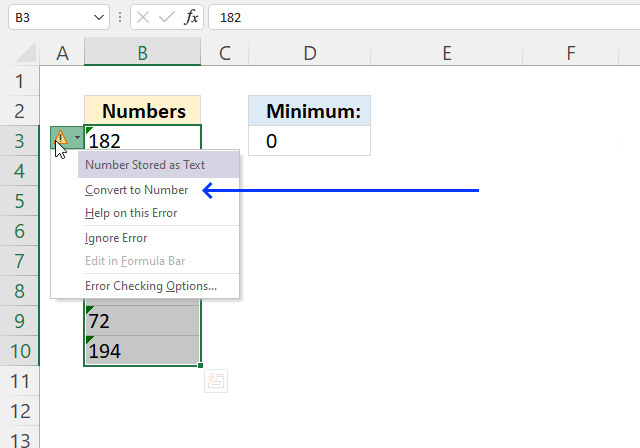4. Press with left mouse button on "Convert to Number".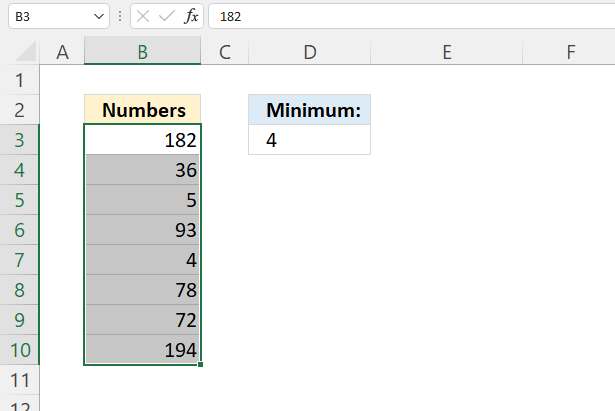The MIN function is now working.

## 7. MIN function ignore errors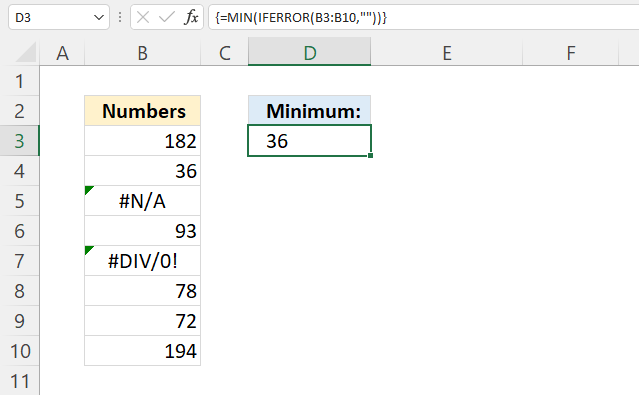The formula in cell D3 extracts the smallest number in cell range B3:B10 ignoring error values.

Array formula in cell D3:

=MIN(IFERROR(B3:B10,""))

How to enter an array formula

### 7.1 Explaining formula

#### Step 1 - Replace error values with nothing

The IFERROR function introduced in Excel 2007 lets you handle most errors in Excel formulas.

IFERROR(valuevalue_if_error)

IFERROR(B3:B10,"")

becomes

IFERROR({182; 36; #N/A; 93; #DIV/0!; 78; 72; 194})

and returns

{182; 36; ""; 93; ""; 78; 72; 194}

#### Step 2 - Extract smallest number

MIN(IFERROR(B3:B10,""))

becomes

MIN({182; 36; ""; 93; ""; 78; 72; 194})

and returns 36.

## 8. MIN function ignoring negative numbers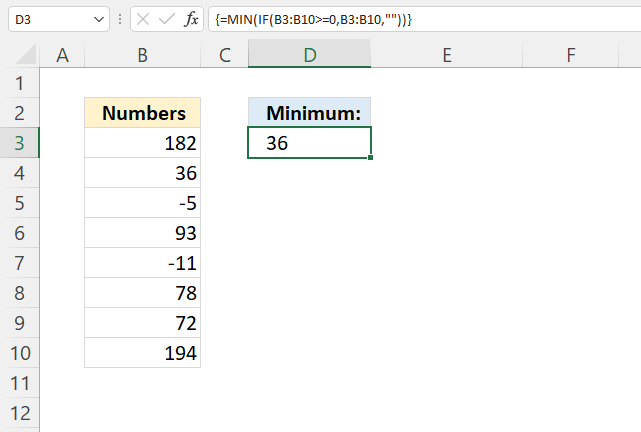The array formula in cell D3 extracts the smallest number in cell range B3:B10 if equal to or larger than zero.

Array formula in cell D3:

=MIN(IF(B3:B10>=0,B3:B10,""))

How to enter an array formula

### Explaining formula

#### Step 1 - Filter out negative numbers

The larger than and the equal sign lets you identify numbers larger than or equal to zero, the result is a boolean value TRUE or FALSE.

B3:B10>=0

becomes

{182; 36; -5; 93; -11; 78; 72; 194}>=0

and returns

{TRUE; TRUE; FALSE; TRUE; FALSE; TRUE; TRUE; TRUE}.

#### Step 2 - Replce TRUE with corresponding number

The IF function returns one value if the logical test is TRUE and another value if the logical test is FALSE.

IF(logical_test, [value_if_true], [value_if_false])

IF(B3:B10>=0, B3:B10, "")

becomes

IF({TRUE; TRUE; FALSE; TRUE; FALSE; TRUE; TRUE; TRUE}, {182; 36; -5; 93; -11; 78; 72; 194}, "")

and returns

{182; 36; ""; 93; ""; 78; 72; 194}.

#### Step 3 - Extract the smallest number

MIN(IF(B3:B10>=0,B3:B10,""))

becomes

MIN({182; 36; ""; 93; ""; 78; 72; 194})

and returns 36.

## 9. MIN function ignore text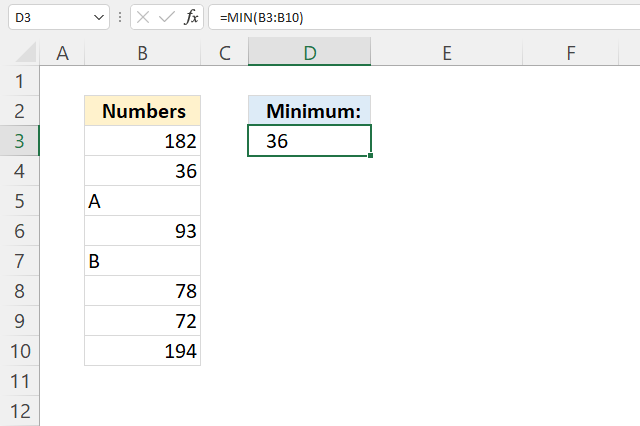The MIN function ignores text values out of the box, there is no need for you to do anything about it. The example above demonstrates two text values in cells B5 and B7, the formula in cell D3 extracts the smallest number in B3:B10 ignoring the text values.

Formula in cell D3:

=MIN(B3:B10)

## 10. MIN function ignore #NA error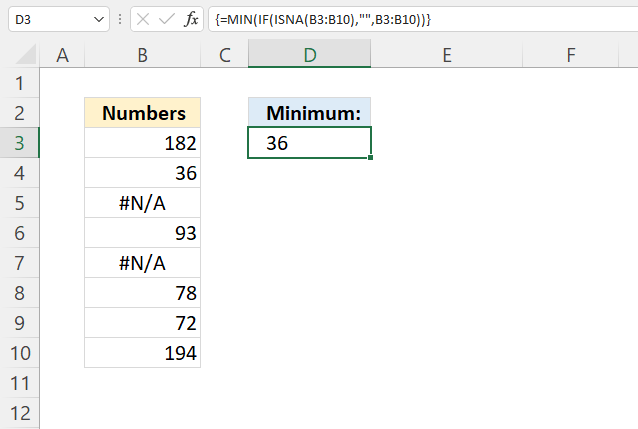Array formula in cell D3:

=MIN(IF(ISNA(B3:B10), "", B3:B10))

How to enter an array formula

### Explaining formula

#### Step 1 - Identify #N/A errors

The ISNA function returns TRUE if a given value is #N/A.

ISNA(value)

ISNA(B3:B10)

becomes

ISNA({182; 36; #N/A; 93; #N/A; 78; 72; 194})

and returns

{FALSE; FALSE; TRUE; FALSE; TRUE; FALSE; FALSE; FALSE}.

#### Step 2 - Replace TRUE with nothing

The IF function returns one value if the logical test is TRUE and another value if the logical test is FALSE.

IF(logical_test, [value_if_true], [value_if_false])

IF(ISNA(B3:B10), "", B3:B10)

becomes

IF({FALSE; FALSE; TRUE; FALSE; TRUE; FALSE; FALSE; FALSE}, "", B3:B10)

becomes

IF({FALSE; FALSE; TRUE; FALSE; TRUE; FALSE; FALSE; FALSE}, "", {182; 36; #N/A; 93; #N/A; 78; 72; 194})

and returns

{182; 36; ""; 93; ""; 78; 72; 194}.

#### Step 3 - Extract minimum number

MIN(IF(ISNA(B3:B10), "", B3:B10))

becomes

MIN({182; 36; ""; 93; ""; 78; 72; 194})

and returns 36.